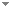Switch to: References

# Game ideals

Annals of Pure and Applied Logic 158 (1-2):23-39 (2009)

 Weak reflection principle, saturation of the nonstationary ideal on ω 1 and diamonds.Víctor Torres-pérez - 2017 - Journal of Symbolic Logic 82 (2):724-736. Direct download (2 more)     Export citationBookmarkThe Magidor function and diamond.Pierre Matet - 2011 - Journal of Symbolic Logic 76 (2):405 - 417.Let κ be a regular uncountable cardinal and λ be a cardinal greater than κ. We show that if 2 <κ ≤ M(κ, λ), then ◇ κ,λ holds, where M(κ, λ) equals $\lambda ^{\aleph }0$ if cf(λ) ≥ κ, and $(\lambda ^{+})^{\aleph _{0}}$ otherwise. Direct download (5 more)     Export citationBookmark5 citations Two-cardinal diamond star.Pierre Matet - 2014 - Mathematical Logic Quarterly 60 (4-5):246-265.No categories Direct download     Export citationBookmark7 citations Non-saturation of the nonstationary ideal on P κ (λ) in case κ≤ cf (λ)< λ.Pierre Matet - 2012 - Archive for Mathematical Logic 51 (3-4):425-432.Given a regular cardinal κ > ω 1 and a cardinal λ with κ ≤ cf (λ) < λ, we show that NS κ,λ | T is not λ+-saturated, where T is the set of all ${a\in P_\kappa (\lambda)}$ such that ${| a | = | a \cap \kappa|}$ and ${{\rm cf} \big( {\rm sup} (a\cap\kappa)\big) = {\rm cf} \big({\rm sup} (a)\big) = \omega}$. Direct download (3 more)     Export citationBookmark1 citation Non‐saturation of the non‐stationary ideal on Pκ (λ) with λ of countable cofinality.Pierre Matet - 2012 - Mathematical Logic Quarterly 58 (1-2):38-45.Given a regular uncountable cardinal κ and a cardinal λ > κ of cofinality ω, we show that the restriction of the non-stationary ideal on Pκ to the set of all a with equation image is not λ++-saturated . We actually prove the stronger result that there is equation image with |Q| = λ++ such that A∩B is a non-cofinal subset of Pκ for any two distinct members A, B of Q, where NGκ, λ denotes the game ideal on Pκ. (...) Direct download     Export citationBookmark3 citations Ideals on $${P_{\kappa}}$$ P κ associated with games of uncountable length.Pierre Matet - 2015 - Archive for Mathematical Logic 54 (3-4):291-328.We study normal ideals on Pκ\documentclass[12pt]{minimal} \usepackage{amsmath} \usepackage{wasysym} \usepackage{amsfonts} \usepackage{amssymb} \usepackage{amsbsy} \usepackage{mathrsfs} \usepackage{upgreek} \setlength{\oddsidemargin}{-69pt} \begin{document}$${P_{\kappa} }$$\end{document} that are defined in terms of games of uncountable length. Direct download (2 more)     Export citationBookmark6 citations Guessing more sets.Pierre Matet - 2015 - Annals of Pure and Applied Logic 166 (10):953-990. Direct download (3 more)     Export citationBookmark6 citations Applications of Pcf Theory to the Study of Ideals On.Pierre Matet - 2022 - Journal of Symbolic Logic 87 (3):967-994.Let $\kappa$ be a regular uncountable cardinal, and a cardinal greater than or equal to $\kappa$. Revisiting a celebrated result of Shelah, we show that if is close to $\kappa$ and (= the least size of a cofinal subset of ) is greater than, then can be represented (in the sense of pcf theory) as a pseudopower. This can be used to obtain optimal results concerning the splitting problem. For example we show that if and, then no (...) Direct download (2 more)     Export citationBookmark1 citation